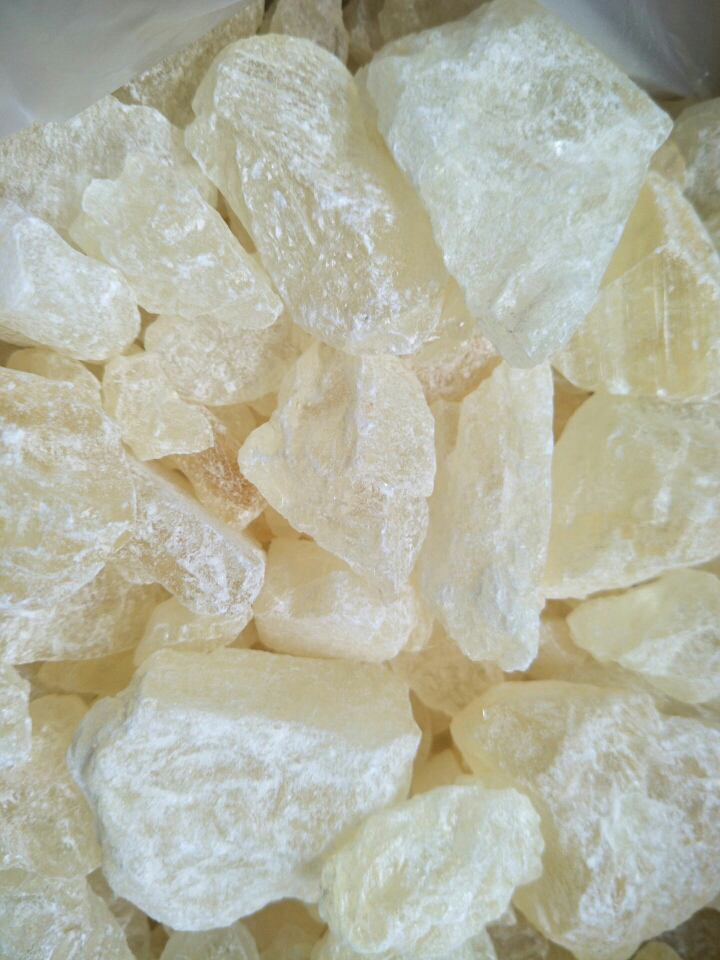# Reconstructing a magnetic grinder using ordinary lathe

The application of magnetic grinding technology in our country is not yet popularized. The key to popularizing and applying this process lies in the research and development of practical machine tools (magnetic grinders). This paper describes the structure principle, technical parameters, and magnetic sensor design and calculation methods of a magnetic lapping machine (device) modified with a common lathe.1. Electrical device 2. Additional pad 3. Centripetal bearing 4. Flywheel 5. Bearing 6. Additional mandrel 7. Solenoid 8. Rear tip 9. Rear tailstock 10. Additional pad 11. Handwheel 12. Electromagnetic Induction 13. Drive Belt 14. Motor
Figure 1 Lathe modified magnetic grinder structure1. Base plate 2. Sliding base 3. Magnetic conductor 4. Solenoid coil 5. Pole head 8. Feed plate 9. Hand wheel 11. Screw
Figure 2 electromagnetic sensor structureFigure 3 magnetic conductor size of the magnetic circuitFigure 4 magnetic sensor rectangular coil size

Assume that the magnetic flux completely passes through the magnetic circuit cross section (without considering magnetic flux leakage), all air gap media are air, and the air gap at the joint is zero. The magnetic conductor size of the magnetic circuit is shown in Figure 3. Work clearance area S = mA = 8 Ã— 230 = 1.84 Ã— 10-3m2, where: pole head width A = 230mm, the pole head surrounded by the workpiece part of the chord length m = 8mm, the working gap is 2mm, the steel saturation magnetic induction B = 2T The magnetic flux of the working gap F=BS=2Ã—1.84Ã—10-3=3.68Ã—10-3Wb. The dimensions of each part of the magnetic conductor are shown in Fig. 3: L1 = 25mm, L2 = 165mm, L3 = 190mm, L4 = 395mm; the sectional size of the magnetic conductor is 230mm Ã— 30mm; the diameter of the workpiece is F11mm. The magnetic potential and the total magnetic potential of the magnetic circuit are calculated as follows: Magnetic field strength in the working gap: H0=B/Î¼=2/(4pÃ—10-7)=0.1595Ã—107A/M
The magnetic potential in the working gap: F0=2H0L0=2Ã—0.1595Ã—107Ã—2Ã—10-3=6400A Magnetic pole area: S=(m+30)A/2=4.37Ã—10-3m2
Magnetic induction of pole head: B=F/S=3.68Ã—10-3/4.37Ã—10-3=0.84T
Magnet head magnetic field strength: H = 940A/m (detected from the magnetization curve of the steel)
The magnetic pole head magnetic potential: F1 = 2HL1 = 2 Ã— 0.94 Ã— 103 Ã— 25 Ã— 10-3 = 47A The remaining magnetic conductor cross-sectional area: S = 30 Ã— 230 Ã— 10-6 = 6.9 Ã— 10-3m2
Magnetic induction of the remaining magnetic conductor: B=3.68Ã—10-3/6.9Ã—10-3=0.534T
The magnetic field strength of the remaining magnetic conductors: H = 680A/m (determined from the magnetization curve of the steel) The magnetic potentials of the magnetic conductors L2, L3, L4: F2, 3, 4 = 2HL2+2HL3 + 2HL4 = 683.4A The sectional area of â€‹â€‹the workpiece : S=8Ã—230Ã—10-3m2 Magnetic induction of the workpiece: B=3.68/1.84=2T Magnetic field strength of the workpiece: H=50000A/m (found from the magnetization curve of 45 steel) The magnetic potential of the workpiece: F=50000 Ã—11Ã—10-3=550A Total magnetic potential of all magnetic sections: F total = F0 + F1 + F2, 3, 4 + F = 7680A Calculated magnetic sensor coil After finding the magnetic potential of the magnetic circuit, calculate it. Magnetic sensor coil parameters. Calculate the wire diameter d Magnetic sensor rectangular coil size shown in Figure 4. The wire diameter d (mm) is calculated as d=0.2(rqINLP/pCU)1â„2 (1) where: rq is the wire specific resistance at qÂ°C, rq=0.016 (1+q/234.5); IN=F is Magnetic potential (A, ampere); LP is the average length of the coil (cm); C is the coefficient considering the voltage drop, C = 0.85. Take the voltage U=220V into the coil and rq=0.0196 when the coil temperature q=50Â°C. From Figure 4, the average coil length LP = 760mm can be calculated. From formula 1, the diameter of the wire can be calculated as d=0.88mm and rounded to 1mm. Calculate the number of turns of the coil N The number of turns of the inductor N is calculated as N=4fLeÃ—102/pd2 (2) Where: L and e are the length and width (cm) of the coil, respectively; d is the diameter of the bare wire (mm) f is the fill factor, f = SK / dJ (dJ + âˆ† / m), where: S is the cross-sectional area of â€‹â€‹the wire, S = pd2/4 (mm2); âˆ† for the thickness of the liner, take âˆ† = 0.05mm; m lining The number of wire layers between the paper, m = 1; K is the coefficient, when the wire diameter d> 0.5, K = 1. Taking the diameter of the coated wire dJ=1.23mm, f=0.5 can be calculated. From equation (2), N = 3846 åŒ can be calculated. The total number of turns of the two coils is 7692 åŒ. According to the need, the technical parameters such as the resistance of the magnetic sensor coil, the power consumption, the electromagnetic suction force, the copper consumption, and the temperature rise of the coil can also be calculated.

Ambrette
Scientific Name(s): Abelmoschus moschatus L. (Medic.)
Common Name(s): Ambrette, Musk Ambrette, Musk okra, Muskdana, Muskmallow

Use

Ambrette has been used as a stimulant and as treatment for a variety of ills, from stomach cancer to hysteria. It is commonly used to scent cosmetics and to flavor foods and drinks. Traditionally, it has been used for treating headaches, cramps, muscular aches and pains, nervous system disorders including depression, and bacterial pathologies.Raw Musk Ambrette

Raw Musk Ambrette,Material Ambrette Musk,Good Quality Musk,Raw Material Musk

Gan Su Original Flavor Co.,ltd , https://www.muskxylol.nl

Posted on